# Completing the Square Parabolas

Remember the pattern for parabolas:

vertical: y=a(x-h)2+k     horizonal: x=a(y-k)2+h

Often parabolas are already listed in this format, but sometimes they are not. In this case, you must put them into the graphing format by completing the square.

To complete the square for a parabola, follow these steps:

1. Identify which variable is squared. Get both terms with that variable on one side of the equation and everything else on the other side.
2. Factor out the squared term's constant.
3. Take the number in front of the first degree term, divide it by two, and square it.
4. Add that number's value to both sides. (Be careful: you have to add the full distributed value.)
5. Factor the resulting trinomial.
6. Move the constant to the other side.
7. Simplify and rearrange if necessary

Examples: Rearrange these equations to match the graphing pattern.
1. y =(2x)2-12x+23
Our squared term is x, so we need the two terms with an x on one side and everything else on the other. That means we need to move the 23.
y - 23 =(2x)2-12x
Next, we need to factor out the squared term's coefficient: 2
y - 23= 2(x2-6x)
We'll now take the number in front of the first degree term: -6. Divide it by 2 (this gives us -3) and square it. This gives us 9.
We need to add this number's value to both sides. But this is more complex than it looks. We must add the 9 inside the parenthesis. This means that we are actually adding 18 to the right hand side (because the 2 would be distributed to the 9). Thus, we must add 18 to the left hand side.
y-23+18=2(x2-6x+9)
Now we'll factor the trinomial in parenthesis:
y - 5 = 2(x-3)(x-3)
And rewrite it:
y - 5 = 2(x-3)2
Now we'll move the constant term (-5) back to the other side.
y = 2(x-3)2+5
This now matches the graphing pattern and allows us to find all our key information.

2.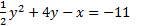Our squared term is y, so we need the two terms with an y on one side and everything else on the other. That means we need to move the -x. Let's also switch the sides of the equation.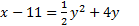Next, we need to factor out the squared term's coefficient: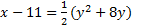We'll now take the number in front of the first degree term: 8. Divide it by 2 (this gives us 4) and square it. This gives us 16.

We need to add this number's value to both sides. But remember we have to be careful. We must add the 16 inside the parenthesis. This means that we are actually adding only 8 to the right hand side (because the 1/2 would be distributed to the 16). Thus, we must add 8 to the left hand side.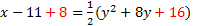Now we'll factor the trinomial in parenthesis: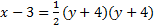And rewrite it: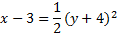Now we'll move the constant term (-3) back to the other side.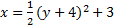This now matches the graphing pattern and allows us to find all our key information.

Practice: Complete the square to make each equation match the graphing pattern:
1. y = 3x2-6x+5
2. x = -2y2-16y-37
3.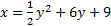4.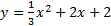5. y = -5x2+20x-17

Answers: 1) y = 3(x-1)2+2   2) x = -2(y+4)2-5    3)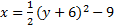4)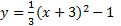5) y = -5(x-2)2+3

 Related Links: Math Fractions Factors